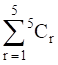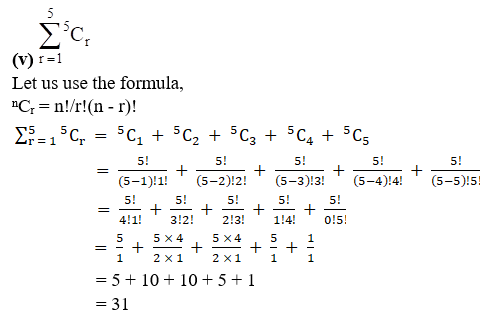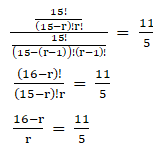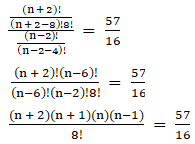• +91 9971497814
• info@interviewmaterial.com

# RD Chapter 17- Combinations Ex-17.1 Interview Questions Answers

### Related Subjects

Question 1 :

Evaluate the following:

(i) 14C3

(ii) 12C10

(iii) 35C35

(iv) n+1Cn

(v)(i) 14C3

Let us use theformula,

nCr = n!/r!(n – r)!

So now, value of n =14 and r = 3

nCr = n!/r!(n – r)!

14C3 = 14! / 3!(14 – 3)!

= 14! / (3! 11!)

= [14×13×12×11!] / (3!11!)

= [14×13×12] / (3×2)

= 14×13×2

= 364

(ii) 12C10

Let us use theformula,

nCr = n!/r!(n – r)!

So now, value of n =12 and r = 10

nCr = n!/r!(n – r)!

12C10 = 12! / 10!(12 – 10)!

= 12! / (10! 2!)

= [12×11×10!] / (10!2!)

= [12×11] / (2)

= 6×11

= 66

(iii) 35C35

Let us use theformula,

nCr = n!/r!(n – r)!

So now, value of n =35 and r = 35

nCr = n!/r!(n – r)!

35C35 = 35! / 35!(35 – 35)!

= 35! / (35! 0!)[Since, 0! = 1]

= 1

(iv) n+1Cn

Let us use theformula,

nCr = n!/r!(n – r)!

So now, value of n =n+1 and r = n

nCr = n!/r!(n – r)!

n+1Cn = (n+1)! / n!(n+1 – n)!

= (n+1)! / n!(1!)

= (n + 1) / 1

= n + 1Question 2 :

If nC12 = nC5,find the value of n.

We know that if nCp = nCq,then one of the following conditions need to be satisfied:

(i) p = q

(ii) n = p + q

So from thequestion nC12 = nC5,we can say that

12 ≠ 5

So, the condition (ii)must be satisfied,

n = 12 + 5

n = 17

The value of nis 17.

Question 3 : If nC4 = nC6,find 12Cn.

We know that if nCp = nCq,then one of the following conditions need to be satisfied:

(i) p = q

(ii) n = p + q

So from thequestion nC4 = nC6,we can say that

4 ≠ 6

So, the condition (ii)must be satisfied,

n = 4 + 6

n = 10

Now, we need tofind 12Cn,

We know the value of nso, 12Cn = 12C10

Let us use theformula,

nCr = n!/r!(n – r)!

So now, value of n =12 and r = 10

nCr = n!/r!(n – r)!

12C10 = 12! / 10!(12 – 10)!

= 12! / (10! 2!)

= [12×11×10!] / (10!2!)

= [12×11] / (2)

= 6×11

= 66

The valueof 12C10 = 66.

Question 4 :

If nC10 = nC12,find 23Cn.

We know that if nCp = nCq,then one of the following conditions need to be satisfied:

(i) p = q

(ii) n = p + q

So from thequestion nC10 = nC12,we can say that

10 ≠ 12

So, the condition (ii)must be satisfied,

n = 10 + 12

n = 22

Now, we need tofind 23Cn,

We know the value of nso, 23Cn = 23C22

Let us use theformula,

nCr = n!/r!(n – r)!

So now, value of n =23 and r = 22

nCr = n!/r!(n – r)!

23C22 = 23! / 22!(23 – 22)!

= 23! / (22! 1!)

= [23×22!] / (22!)

= 23

The valueof 23C22 = 23.

Question 5 :

If 24Cx = 24C2x + 3,find x.

We know that if nCp = nCq,then one of the following conditions need to be satisfied:

(i) p = q

(ii) n = p + q

So from thequestion 24Cx = 24C2x + 3,we can say that

Let us check forcondition (i)

x = 2x + 3

2x – x = -3

x = -3

We know that for acombination nCr, r≥0, r should be a positive integerwhich is not satisfied here,

So, the condition (ii)must be satisfied,

24 = x + 2x + 3

3x = 21

x = 21/3

x = 7

The value of xis 7.

Question 6 :

If 18Cx = 18Cx + 2,find x.

We know that if nCp = nCq,then one of the following conditions need to be satisfied:

(i) p = q

(ii) n = p + q

So from thequestion 18Cx = 18Cx + 2,we can say that

x ≠ x + 2

So, the condition (ii)must be satisfied,

18 = x + x + 2

18 = 2x + 2

2x = 18 – 2

2x = 16

x = 16/2

= 8

The value of xis 8.

Question 7 :

If 15C3r = 15Cr + 3,find r.

We know that if nCp = nCq,then one of the following conditions need to be satisfied:

(i) p = q

(ii) n = p + q

So from thequestion 15C3r = 15Cr + 3,we can say that

Let us check forcondition (i)

3r = r + 3

3r – r = 3

2r = 3

r = 3/2

We know that for acombination nCr, r≥0, r should be a positive integerwhich is not satisfied here,

So, the condition (ii)must be satisfied,

15 = 3r + r + 3

15 – 3 = 4r

4r = 12

r = 12/4

= 3

The value of ris 3.

Question 8 :

If 8Cr – 7C3 = 7C2,find r.

To find r, let usconsider the given expression,

8Cr – 7C3 = 7C2

8Cr = 7C2 + 7C3

We know that nCr + nCr+ 1 = n + 1Cr + 1

8Cr = 7 + 1C2+ 1

8Cr = 8C3

Now, we know thatif nCp = nCq, thenone of the following conditions need to be satisfied:

(i) p = q

(ii) n = p + q

So from thequestion 8Cr = 8C3,we can say that

Let us check forcondition (i)

r = 3

Let us also check forcondition (ii)

8 = 3 + r

r = 5

The values of‘r’ are 3 and 5.

Question 9 :

If 15Cr15Cr – 1 =11: 5, find r.

Given:

15Cr15Cr – 1 =11: 5

15Cr / 15Cr– 1 = 11 / 5

Let us use theformula,

nCr = n!/r!(n – r)!5(16 – r) = 11r

80 – 5r = 11r

80 = 11r + 5r

16r = 80

r = 80/16

= 5

The value of ris 5.

Question 10 :

If n + 2C8n – 2P4 =57: 16, find n.

Given:

n + 2C8n – 2P4 =57: 16

n + 2C8 / n – 2P4 =57 / 16

Let us use theformula,

nCr = n!/r!(n – r)![(n+2)!(n-6)!] / [(n-6)! (n-2)! 8!] = 57/16

(n+2) (n+1) (n) (n-1)/ 8! = 57/16

(n+2) (n+1) (n) (n-1)= (57×8!) / 16

(n+2) (n+1) (n) (n-1)= [19×3 × 8×7×6×5×4×3×2×1]/16

(n + 2) (n + 1) (n) (n– 1) = 21 × 20 × 19 × 18

Equating thecorresponding terms on both sides we get,

n = 19

The value of nis 19.

Todays Deals### RD Chapter 17- Combinations Ex-17.1 Contributorskrishan

Name:
Email:

# Latest News# 9000 interview questions in different categories